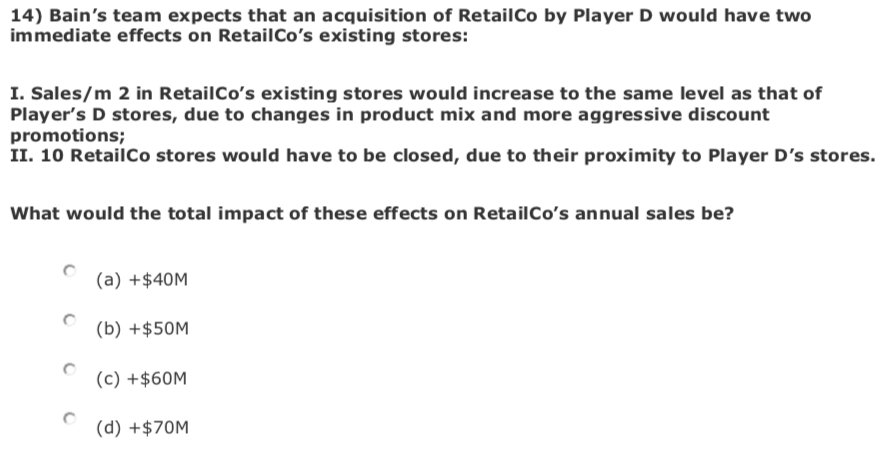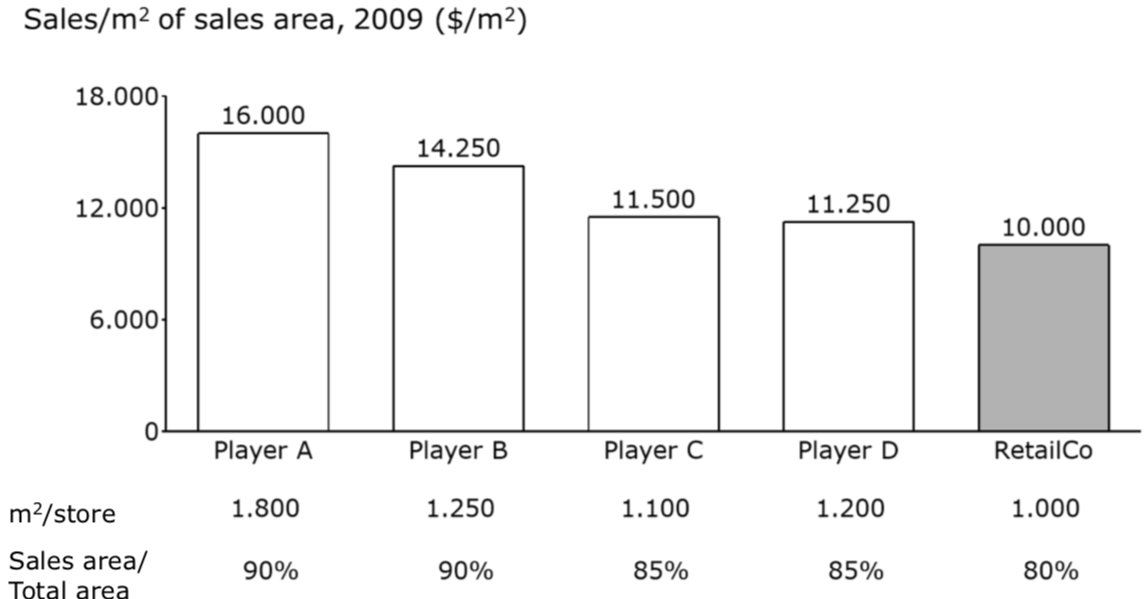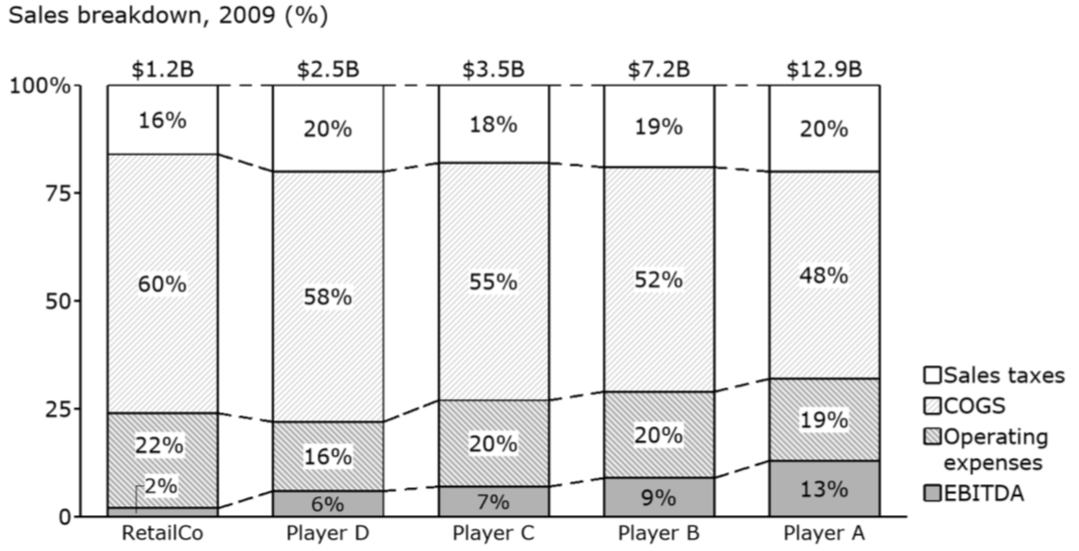# Bain Question - Business Case Sample

Bain Bain & Company business case Math problem Online test Written test
New answer on Jun 04, 2020
1.2 k Views

Hi,

This is a question from Bain sample case. I attach the question and relevant graphs below. Would appreciate if someone can give me hints on how to solve this problem. Thank you very much!• Date ascending
• Date descending

Result is C) +60M\$

1. Identify number of stores of Retail Co

• Sales area= sales : sales/m2 = 1.2bn\$ : 10,000 = 120,000 m2
• Sales area = 80% of store area
• Store area = ((sales area * 100%) : 80%) : m2/store = ((120,000 m2 * 100%) : 80%) : 1,000 m2 = 150

2. Decrease of number of stores by 10

• Number of stores after acquisition = 140
• Assumption: sales/m2 of RetailCo = 11,250 \$/m2
• Store area (=100%) = number of stores * m2/store = 140 * 1,000 m2 = 140,000 m2
• Sales area = 80% of store area = (140,000 m2 * 80%) : 100% = 112,000 m2
• Sales = sales area * new sales/m2 = 112,000 m2 * 11,250 \$/m2 = 1.26bn \$

3. Increase in sales

• Old sales: 1.2bn\$
• New sales: 1.26bn\$
• Increase by: 60M\$

Hello!

Don´t let them overwhelm you with graphs!

It´s only a way to dress in a fancy way simple data.

1. Sales/store= 10 000 * 1000 * 80% = 8M USD/ store
2. Number of stores = 1.2 Bn / 8 M = 150 total stores
3. 11 250 * 1000 * 80% * 140 - 1.2 Bn= 60M USD

Hope it helps!

Cheers,

Clara

(edited)

Hi there,

Give the others have already answered the question for you, let me give you three tips on how to nail this question next time :)

1) Question first - read the immediate question first (i.e. total impact of effects on annual sales). Skip the context, skip the charts, read the one sentence that asks you the question that matters. This helps you frame your mindset and pull out only the information that matters.

2) Block out the charts - think about what information you need before you read the charts. What #s would you need and how would you use/multiply them? Again, this keeps you focused on what actually matters once you look at the charts.

3) Answer Plug-in/Elimination - Read the answers early on. First, quickly figure out which ones clearly don't make sense (and cross them out). Second, plug and play the answers they've provided. In some cases you can back-solve the answer. To summarize: Test the answers provided instead of doing all the work to find the answer!

Hi,

I did a quick calculation:

\$/store= 10 000 * 1000* 80% = 8 mio \$/ store

#stores = 1.2 bn / 8 mio = 150

(11 250 * 1000 * 80% * 140 ) - 1.2 bn \$ = +60 mio \$